International
Tables for
Crystallography
Volume B
Reciprocal space
Edited by U. Shmueli

International Tables for Crystallography (2010). Vol. B, ch. 1.3, pp. 70-71   | 1 | 2 |

## Section 1.3.4.2.2.3. Classification of crystallographic groups

G. Bricognea

aGlobal Phasing Ltd, Sheraton House, Suites 14–16, Castle Park, Cambridge CB3 0AX, England, and LURE, Bâtiment 209D, Université Paris-Sud, 91405 Orsay, France

#### 1.3.4.2.2.3. Classification of crystallographic groups

| top | pdf |

Let Γ be a crystallographic group, Λ the normal subgroup of its lattice translations, and G the finite factor group. Then G acts on Λ by conjugation [Section 1.3.4.2.2.2(d)] and this action, being a mapping of a lattice into itself, is representable by matrices with integer entries.

The classification of crystallographic groups proceeds from this observation in the following three steps:

 Step 1: find all possible finite abstract groups G which can be represented byinteger matrices. Step 2: for each such G find all its inequivalent representations byinteger matrices, equivalence being defined by a change of primitive lattice basis (i.e. conjugation by ainteger matrix with determinant ±1). Step 3: for each G and each equivalence class of integral representations of G, find all inequivalent extensions of the action of G from Λ to, equivalence being defined by an affine coordinate change [i.e. conjugation by an element of].

Step 1leads to the following groups, listed in association with the crystal system to which they later give rise: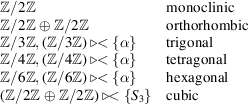and the extension of these groups by a centre of inversion. In this list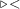denotes a semi-direct product [Section 1.3.4.2.2.2(d)], α denotes the automorphism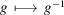, and(the group of permutations on three letters) operates by permuting the copies of(using the subgroupof cyclic permutations gives the tetrahedral subsystem).

Step 2leads to a list of 73 equivalence classes called arithmetic classes of representations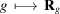, whereis ainteger matrix, withand. This enumeration is more familiar if equivalence is relaxed so as to allow conjugation by rationalmatrices with determinant ± 1: this leads to the 32 crystal classes. The difference between an arithmetic class and its rational class resides in the choice of a lattice mode. Arithmetic classes always refer to a primitive lattice, but may use inequivalent integral representations for a given geometric symmetry element; while crystallographers prefer to change over to a nonprimitive lattice, if necessary, in order to preserve the same integral representation for a given geometric symmetry element. The matrices P anddescribing the changes of basis between primitive and centred lattices are listed in Table 5.1.3.1and illustrated in Figs. 5.1.3.2to 5.1.3.8, pp. 80–85, of Volume A of International Tables (Arnold, 2005).

Step 3gives rise to a system of congruences for the systems of nonprimitive translationswhich may be associated to the matricesof a given arithmetic class, namely:first derived by Frobenius (1911). If equivalence under the action ofis taken into account, 219 classes are found. If equivalence is defined with respect to the action of the subgroupofconsisting only of transformations with determinant +1, then 230 classes called space-group types are obtained. In particular, associating to each of the 73 arithmetic classes a trivial set of nonprimitive translationsyields the 73 symmorphic space groups. This third step may also be treated as an abstract problem concerning group extensions, using cohomological methods [Ascher & Janner (1965); see Janssen (1973)for a summary]; the connection with Frobenius's approach, as generalized by Zassenhaus (1948), is examined in Ascher & Janner (1968).

The finiteness of the number of space-group types in dimension 3 was shown by Bieberbach (1912) to be the case in arbitrary dimension. The reader interested in N-dimensional space-group theory for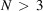may consult Brown (1969), Brown et al. (1978), Schwarzenberger (1980) and Engel (1986). The standard reference for integral representation theory is Curtis & Reiner (1962).

All three-dimensional space groups G have the property of being solvable, i.e. that there exists a chain of subgroups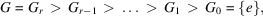where eachis a normal subgroup ofand the factor groupis a cyclic group of some order. This property may be established by inspection, or deduced from a famous theorem of Burnside [see Burnside (1911), pp. 322–323] according to which any group G such that, with p and q distinct primes, is solvable; in the case at hand,and. The whole classification of 3D space groups can be performed swiftly by a judicious use of the solvability property (L. Auslander, personal communication).

Solvability facilitates the indexing of elements of G in terms of generators and relations (Coxeter & Moser, 1972; Magnus et al., 1976) for the purpose of calculation. By definition of solvability, elementsmay be chosen in such a way that the cyclic factor groupis generated by the coset. The setis then a system of generators for G such that the defining relations [see Brown et al. (1978), pp. 26–27] have the particularly simple form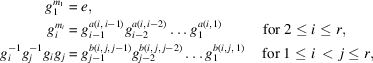with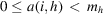and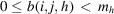. Each element g of G may then be obtained uniquely as an `ordered word':with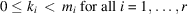, using the algorithm of Jürgensen (1970). Such generating sets and defining relations are tabulated in Brown et al. (1978, pp. 61–76). An alternative list is given in Janssen (1973, Table 4.3, pp. 121–123, and Appendix D, pp. 262–271).

### References

Arnold, H. (2005). Transformations in crystallography. In International Tables for Crystallography, Vol. A. Space-Group Symmetry, edited by Th. Hahn, Chapter 5.1. Heidelberg: Springer.
Ascher, E. & Janner, A. (1965). Algebraic aspects of crystallography. I. Space groups as extensions. Helv. Phys. Acta, 38, 551–572.
Ascher, E. & Janner, A. (1968). Algebraic aspects of crystallography. II. Non-primitive translations in space groups. Commun. Math. Phys. 11, 138–167.
Bieberbach, L. (1912). Über die Bewegungsgruppen der Euklidischen Raume II. Math. Ann. 72, 400–412.
Brown, H. (1969). An algorithm for the determination of space groups. Math. Comput. 23, 499–514.
Brown, H., Bülow, R., Neubüser, J., Wondratschek, H. & Zassenhaus, H. (1978). Crystallographic Groups of Four-Dimensional Space. New York: John Wiley.
Burnside, W. (1911). Theory of Groups of Finite Order, 2nd ed. Cambridge University Press.
Coxeter, H. S. M. & Moser, W. O. J. (1972). Generators and Relations for Discrete Groups, 3rd ed. Berlin: Springer-Verlag.
Curtis, C. W. & Reiner, I. (1962). Representation Theory of Finite Groups and Associative Algebras. New York: Wiley–Interscience.
Engel, P. (1986). Geometric Crystallography. Dordrecht: Kluwer Academic Publishers.
Frobenius, G. (1911). Über die unzerlegbaren diskreten Bewegungsgruppen. Sitzungsber. Preuss. Akad. Wiss. Berlin, 29, 654–665.
Janssen, T. (1973). Crystallographic Groups. Amsterdam: North-Holland.
Jürgensen, H. (1970). Calculation with the elements of a finite group given by generators and defining relations. In Computational Problems in Abstract Algebra, edited by J. Leech, pp. 47–57. Oxford: Pergamon Press.
Magnus, W., Karrass, A. & Solitar, D. (1976). Combinatorial Group Theory: Presentations of Groups in Terms of Generators and Relations, 2nd revised ed. New York: Dover Publications.
Schwarzenberger, R. L. E. (1980). N-Dimensional Crystallography. Research Notes in Mathematics, Vol. 41. London: Pitman.
Zassenhaus, H. (1948). Über eine Algorithmus zur Bestimmung der Raumgruppen. Commun. Helv. Math. 21, 117–141.